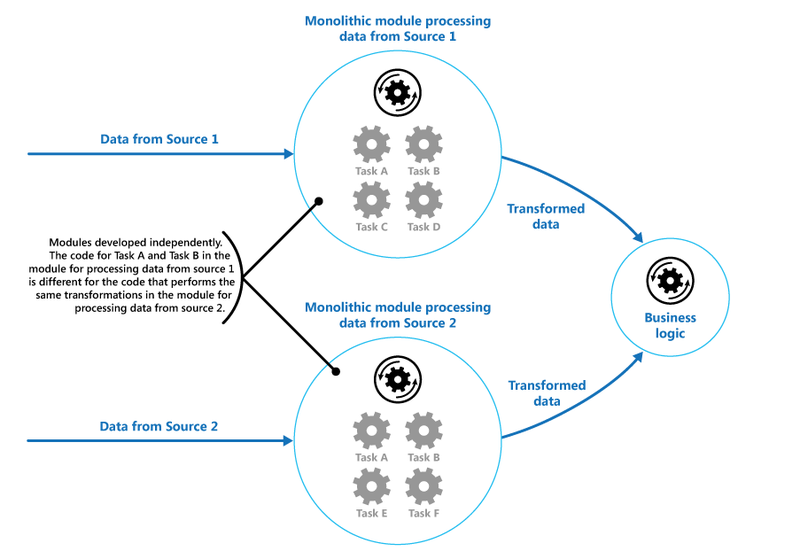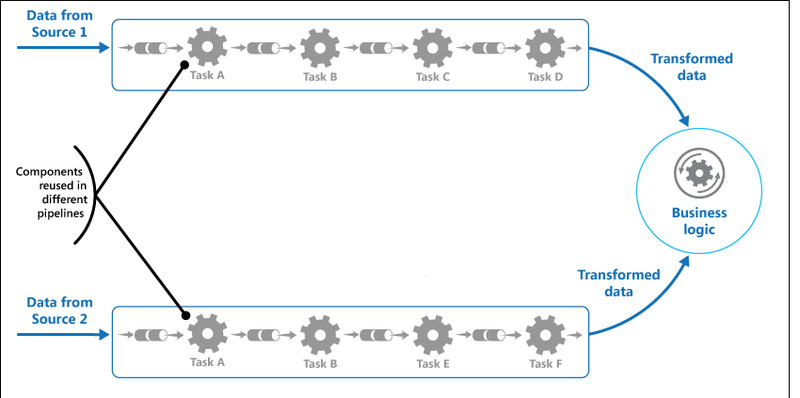# Pipe & Filter Pattern in Python

Dec. 11, 2020

Kyle Kaniecki

Edit 1 (12/19/2020): I changed how filters placed their content and defaulted the base content filter to place its content at cell (1, 1). This allowed me to implement vertical and horizontal stacking based on the previous blocks content size. I added an example of that class below, along with the base content filter I implemented.

Edit 2 (12/30/2020): I added the code for my vertical stack filter at the end of the article

I was recently given a contracting job to redesign how a contracting website generates documents from the data that was given inside it's databases. One example was a customer invoice document, which required taking a job's materials list and generating an invoice for a customer given the material sale price and quantity. Another requirement was a purchase order which could include things like a vendor id and header included in the document. They are solid requirements, but a little difficult to model once you get down down into the weeds of the modeling.

Also, to add some context, the app already had document functionality implemented using openpyxl, so I needed to use their model of modifying excel spreadsheets. And while openpyxl has some great tools to manipulate excel spreadsheets, it didn't have a great way to implement grouped data. Cell manipulation was largely point and edit cells in a singular type of fashion.

After some research, I decided on a pipeline-like pattern, where documents would flow through a pipeline of filters (think Instagram filters) that would apply content or modify the excel sheet's appearance based on a job. Each filter would be responsible for exactly one action, applying a group of data to a worksheet, almost like a stamp of sorts.

## So, What is the Pipe & Filter pattern?**taken shamelessly from Microsoft, don't sue me

This image is great at showing a naïve solution to our document problem! We would be feeding each "document" model some data from a job, maybe with a vendor or materials list, applying some jobs to it, but then process the documents exactly the same at the end. However, we then quickly run into a problem once we start creating these big monolithic processes. Once we finalize one, we only need to change it a little for a new type of document (swap C&D for E&F). But because they are monolithic, we would mostly likely end up copying & pasting code around. Not a good idea. We should be applying DRY principles; writing our code once and reusing it where necessary. So let's take a look at a better design pattern:What are the differences? Well, firstly we can see that the input of each task has changed from before. Instead of getting the data directly from the source, they are getting the output of the previous task. In our case, this would be a document from the task before it. However, now our tasks are much more modular and do not have implicit dependencies on each other like the order of execution. They just run one task and do it well and pass their result to the next thing. Plus, now if we want to create a new document model in our app, we simply define an ordered list of tasks to run and things work as we expect them to. We could even create a pipeline at runtime if we wanted to by switching out parts and/or adding others.

Now that we have the design, let's dig into some example code.

```from __future__ import annotations

from abc import abstractmethod

from openpyxl.worksheet.worksheet import Worksheet

class BaseWorksheetFilter:
"""
https://docs.microsoft.com/en-us/azure/architecture/patterns/pipes-and-filters
"""

def __init__(self, position: (int, int) = (1, 1)):
self.position = position

def set_position(self, pos: (int, int)):
self.position = pos

@abstractmethod
def __call__(self, worksheet: Worksheet) -> Worksheet:
return worksheet
```

Here was my abstract base filter class that defines how filters should look and behave to other third party objects. Nothing too exciting here. A couple of quick notes:

1. We use the abstract method decorator to ensure all concrete classes implement the call method. This ensures we don't have some wonky class mixing call happening.
2. Our call method takes in a worksheet, does some work on it, and then outputs that same worksheet instance

Okay, now that we know what our tasks will look like, it's time to build our pipeline class that puts all of these tasks together in some order.

```class WorksheetPipeline:
def __init__(self, worksheet: Optional[Worksheet] = None):
self.worksheet = worksheet or Workbook().active
self.filters = []

def pipe(self, *filters: Iterable[BaseWorksheetFilter]):
self.filters = self.filters + list(filters)
return self

def execute(self):
for worksheet_filter in self.filters:
worksheet_filter(self.worksheet)

def set_title(self, title: str):
self.worksheet.title = title
```

Nice, so let's go over what this class is doing.

#### The .pipe() method

This method is responsible for taking in some iterable of filters and appending them to our collection of filters in this pipeline. I decided to go with python's extended iterable unpacking functionality since it modeled rxjs's API, and I liked the pattern.

#### The .execute() method

This method is responsible for actually calling all of our accumulated filters and applying them to our document.

So together, this will allow us to apply some pipeline of filters to our openpyxl worksheet. Awesome. Now let's show a few concrete classes that I created, and an example of the benefits and usage of this design.

```class HideGridlinesFilter(BaseWorksheetFilter):
def __call__(self, worksheet):
worksheet.sheet_view.showGridLines = False
return worksheet
```

A basic filter that just hides the gridlines of an excel worksheet

```@dataclass
class TransitionsCellContent:

value: str = ""
font: str = "Calibri"
bold: bool = False
horizonal_alignment: Optional[str] = None
vertical_alignment: Optional[str] = None
wrap_text: Optional[bool] = None
number_format: Optional[str] = None
image: Optional[str] = None

def __str__(self):
return str(self.value)
```

A dataclass to represent a cell's content and allow for select rich styling

```class ContentFilter(BaseWorksheetFilter):
def __init__(
self, content: List[List[Union[str, TransitionsCellContent]]] = [], **kwargs
):
super().__init__(**kwargs)
self.content = content

def build_content(self):
return [[]]

def get_dimensions(self) -> (int, int):
if not self.content:
self.content = self.build_content()

return len(self.content), max(self.content, key=lambda row: len(row))

def __call__(self, worksheet):
if not self.content:
self.content = self.build_content()

row_offset, col_offset = self.position
for row_index in range(len(self.content)):
for col_index in range(len(self.content[row_index])):
# Grab the cell and apply our content to it
cell = worksheet.cell(row_offset + row_index, col_offset + col_index)
value = self.content[row_index][col_index]
cell.value = str(value)

# If they passed in rich content, let's apply the styling
if isinstance(value, TransitionsCellContent):
# If it is an image, then let's put the image in tha bitch
if value.image:
img = Image(value.image)
img,
get_cell_string(
row_offset + row_index, col_offset + col_index
),
)
else:
cell.font = Font(name=value.font, bold=value.bold)
cell.alignment = Alignment(
horizontal=value.horizonal_alignment,
vertical=value.vertical_alignment,
wrap_text=value.wrap_text or False,
)

if value.number_format is not None:
cell.number_format = value.number_format

cell.value = value.value

else:
cell.value = str(value)

return worksheet
```

A basic content filter that takes in an arbitraty sized matrix of content

```class JobTotalCostFilter(ContentFilter):
def __init__(self, job: Job, include_remodel=False, **kwargs):
super().__init__(**kwargs)
self.job = job
self.include_remodel = include_remodel

def build_content(self):
mat_total = self.job.products.aggregate(
total=Sum(F("sale_price") * F("quantity"), output_field=FloatField())
)["total"]

sales_tax = calc_sales_tax(mat_total)

total_total = mat_total + sales_tax

if self.include_remodel:
remodel_total = float(
self.job.remodels.aggregate(total=Sum("sale_price"))["total"]
)
total_total += remodel_total

total_cost_row = (
[TransitionsCellContent(value="Total Project:", bold=True)]
+ [""] * 3
+ [
TransitionsCellContent(
value=round(total_total, 2),
bold=True,
number_format='_(\$* #,##0.00_);_(\$* (#,##0.00);_(\$* "-"??_);_(@_)',
)
]
)

return [total_cost_row]
```

A job total cost filter that outputs the total as a row with a label

Sweet! Now we have a couple filters to play around with, lets use them and create some kind of excel spreadsheet. I'll keep the example somewhat small for brevity, but it should illustrate what I'm trying to demonstrate.

```# Create an arbitrary workbook
excel_workbook = Workbook()

# Create our pipeline
pipeline = WorksheetPipeline(excel_workbook.active)

# Pipe in our job cost filter to our
pipeline.pipe(
JobTotalCostFilter((1, 1), job)
)

# If we are printing an estimate, let's add the customer's Address
if is_estimate:
# Example using the content filter for simple strings
pipeline.pipe(
ContentFilterFilter((2, 1), [["This is the estimate subtotal"]])
)
# Otherwise, print the company Header in the content filter
else:
pipeline.pipe(
ContentFilter((3, 1), [["Transitions Kitchen and Bath"]])
)

# Send our worksheet through the pipeline
pipeline.execute()
```

Nice! Now, we can dynamically add filters to a pipeline based on ourside parameters, so our document filters can be organized neatly. In the future, I would add ways to manipulate the pipeline in a more precise fashion, such as inserting in the middle or removing filters. But for now, this is will suffice for my needs.

## Conclusion

Playing around with the pipe and filter pattern was a lot of fun, and really kept me engaged during some of my contract work. Not only will it make my life much easier if I have to do future work on that subsystem, but it will also hopefully allow other developers to easily add functionality as well. I definitely love the low coupling of the pattern, but I did find that some coupling was necessary for some content. Maybe in the future I would add a few improvements:

• Create some kind of GroupFilter that inserts other filters on the page in a vertical sequence and move treat subpipelines as one group. Then, subclasses like VerticalStackFilter and HorizontalStackFilter are possible and would make stacked content much easier
• Add some kind of way to manipulate more than just openpyxl worksheets, since things like Contracts and other documents are better being PDFs than excel sheets.

Edit (12-30-2020)

I've since written a vertical content filter, so I figured I would share it in the blog article below

```class VerticalStack(ContentFilter):
def __init__(self, filters: Iterable[ContentFilter], **kwargs):
super().__init__(**kwargs)
self.filters = list(filters)

def get_dimensions(self) -> (int, int):
max_width = 1
height = 1
for doc_filter in self.filters:
filter_h, filter_w = doc_filter.get_dimensions()

height += filter_h
max_width = max(max_width, filter_w)
return height, max_width

def push(self, content_filter: ContentFilter):
self.filters.append(content_filter)

def pop(self) -> ContentFilter:
return self.filters.pop()

def __call__(self, worksheet):
start_row, start_col = self.position
row_offset = 0

for doc_filter in self.filters:
doc_filter.set_position((start_row + row_offset, start_col))
doc_filter(worksheet)
row_offset += doc_filter.get_dimensions()

return worksheet
```